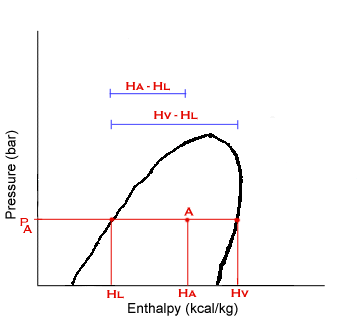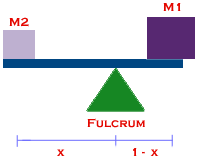LEVER RULEAt Point A:
x = vapor fraction
1-x = liquid fraction

Point A represents a mixture of saturated liquid and vapor at pressure, PA. The enthapy at A, HA, is a weighted average of the enthalpies of the saturated phases:This is the same formula used to balance a seesaw.Hence the name "lever rule." The lever rule can also be used for other properties besides H, like volume.

### Question

At 25°C, the specific volume of saturated liquid is 1.003 cm^3/g and the volume of saturated vapor is 43400 cm^3/g. What is the volume of a mixture with vapor fraction, x=0.3?